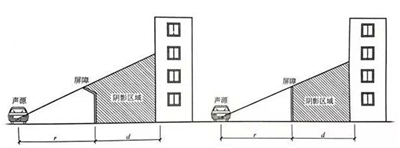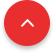# Sound Barrier Principle and Calculation

Feb. 19, 2019
Share:

Sound barrier sound insulation principle:

According to the principle of geometrical acoustics, the sound is transmitted in a straight line outdoors. If a wall or a certain amount of sound is inserted between the sound source and the receiving point to block the sound propagation path, so that the sound source is not visible at the receiving position, no direct sound received, then the sound pressure level at the receiving point will be significantly reduced. This is the principle of sound barrier sound insulation. Sometimes we can't completely close the noise source, it also requires a lower cost to achieve the desired noise reduction effect.

Because sound waves will reflect when they encounter obstacles, so inserting a dense barrier between the source and receiver reduces noise.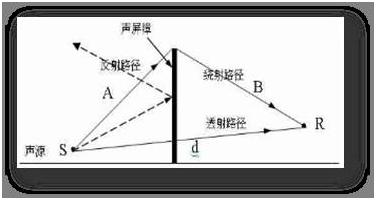Due to the fluctuating nature of the sound source, there is always a part of the sound wave that is diffracted from the top of the sound barrier, which makes the attenuation of the sound barrier limited.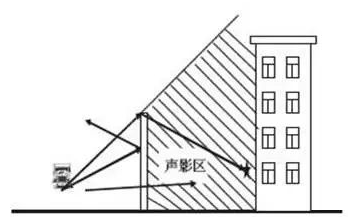Insertion loss and its calculation

There are many vehicles on the road, the picture below shows the spectrum of the bus at a constant speed, the measuring point in the car.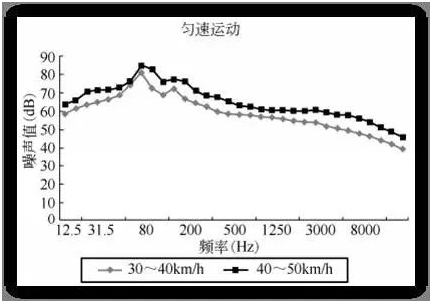Insertion loss is the difference between the sound pressure level received at the receiving point and the sound pressure level when there is no sound barrier after inserting a sound barrier between the sound source and the receiving point.

Considering that the A-weighted spectrum of the car engine is mainly highlighted at 250~2000Hz, therefore the amount of attenuation of the traffic noise A sound level can be roughly determined by the attenuation produced by the sound barrier at 500 Hz.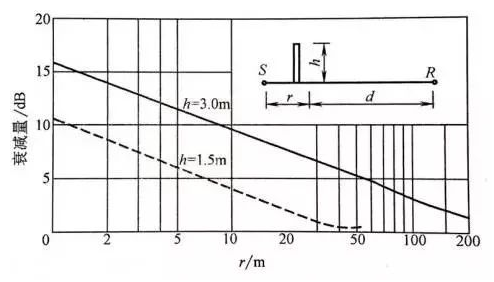Attenuation by placing a h high sound barrier between the observer R and a point source with a frequency of 500 Hz, applicable to the case where d is much larger than r. For example, d=r.

The figure above shows the sound attenuation of the sound barrier height between 1.5m and 3m above the line of sight between the source and the receiver. Line applies to:

The sound source distance r and the receiver distance d are both greater than the barrier height h;

The distance r is much larger than d, or d is much larger than r;

The barrier is thin and the surface is a reflector;

The barrier length is at least 4 times larger than either r or d.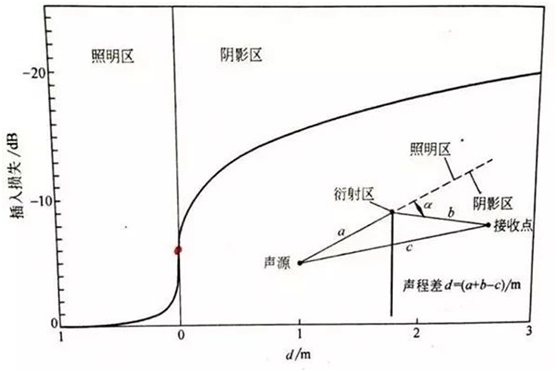Calculation of sound barrier insertion loss

For an infinitely long sound barrier with sufficient sound insulation,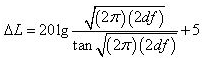In the formula, ΔL is the insertion loss (dB);d is sound path difference, d=a+b-c(m);f is frequency(Hz).

It can be seen from the above formula:

Even if the difference in sound path is small, there also has a 5dB insertion loss.

Theoretically speaking, the greater the insertion loss of the sound barrier, but no more than 24dB.

The role of the sound barrier is more obvious in the sound shadow area, while the sound barrier hardly works in the lighting area.

If not consider the effect of sound transmission from the sound barrier, generally as long as the transmission loss of the sound barrier is 10dB higher than the insertion loss, its impact is negligible. Otherwise it will reduce the sound attenuation of the sound barrier.

It works under conditions where the sound barrier is infinitely long. That is, the sound is only diffracted from the top of the barrier, not diffracting from both sides of the sound barrier. In fact, the sound barrier cannot be infinitely long.

For line source, such as traffic noise, the length of the sound barrier should be: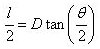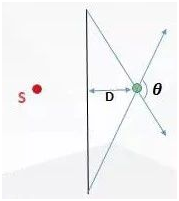In the formula, l is the length of the sound barrier, D is the vertical distance from the receiving point to the sound barrier, θis the angle between the receiving point and the line connecting the two ends of the sound barrier.

There are numerous factors affecting the insertion loss of the sound barrier, Such as the relative position, height and form of the sound barrier, etc.

The position of the sound barrier should be as close as possible to the sound source, this will help expand the sound and shadow area, thereby improving sound attenuation.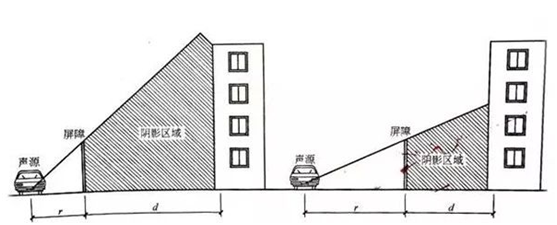Influence of Sound Barrier and Sound Source Level Relative Position on Sound Attenuation

The higher the sound barrier, the larger the shaded area, the greater the attenuation of the sound, this is obvious.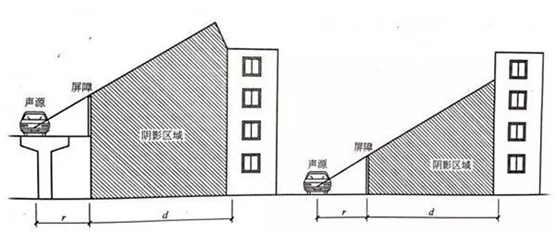Comparison of sound attenuation effects of sound barriers between elevated highways and ground highways

The form of the sound barrier also has a greater impact on sound attenuation.